# Learn Calculus Using Symbolic Math Toolbox

Learn calculus and applied mathematics using the Symbolic Math Toolbox™. This example shows the introductory functions `fplot`, `diff`, and `int`.

To manipulate a variable, create an object of type `syms`.

```disp('Create a symbolic variable for x') disp('>> syms x') syms x ```
```Create a symbolic variable for x >> syms x ```

After a symbolic variable is defined, you can build and visualize functions with `fplot`.

```disp('Build the function f(x) and plot it') disp('>> f(x) = 1/(5+4*cos(x))') disp('>> fplot(f)') f(x) = 1/(5+4*cos(x)) figure; fplot(f) title('Plot of f(x)') ```
```Build the function f(x) and plot it >> f(x) = 1/(5+4*cos(x)) >> fplot(f) f(x) = 1/(4*cos(x) + 5) ```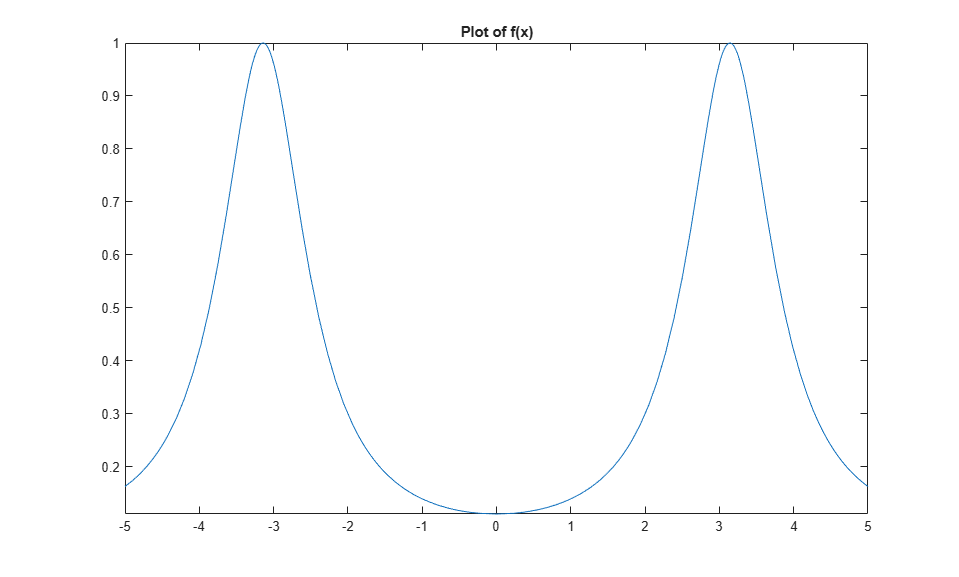Evaluate the function at x = pi/2 using math notation.

```disp('Evaluate f(x) at x = pi/2') disp('>> f(pi/2)') f(pi/2) ```
```Evaluate f(x) at x = pi/2 >> f(pi/2) ans = 1/5 ```

Many functions can work with symbolic variables. For example, the function `diff` differentiates a function.

```disp('Differentiate f(x) and plot the result') disp('>> f1 = diff(f)') disp('>> fplot(f1)') f1 = diff(f) figure; fplot(f1) title("Plot of the derivative of f") ```
```Differentiate f(x) and plot the result >> f1 = diff(f) >> fplot(f1) f1(x) = (4*sin(x))/(4*cos(x) + 5)^2 ```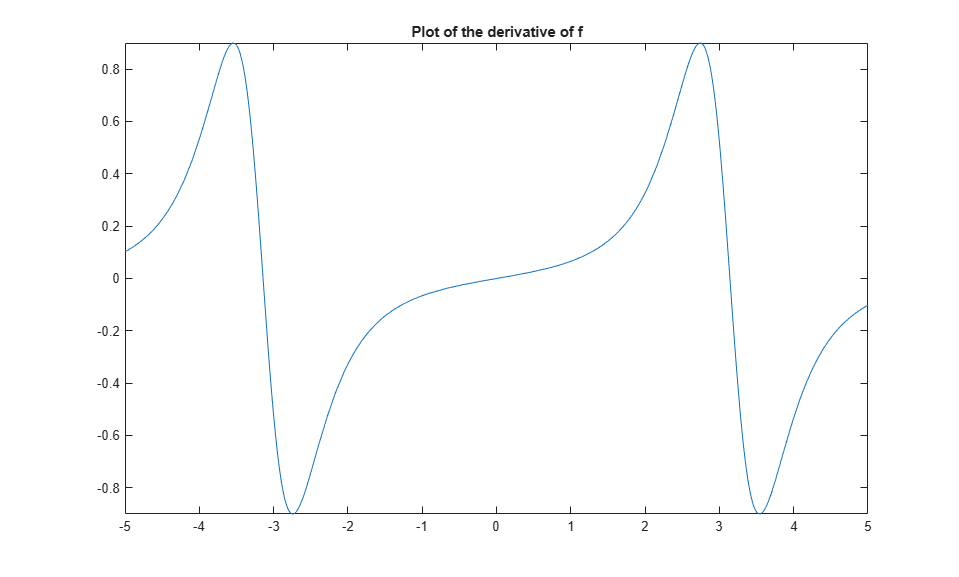The `diff` function can also find the Nth derivative. The next examples shows the second derivative.

```disp('Compute the second derivative of f(x) and plot it') disp('>> f2 = diff(f,2)') disp('>> fplot(f2)') f2 = diff(f,2) figure; fplot(f2) title("Plot of the 2nd derivative of f(x)") ```
```Compute the second derivative of f(x) and plot it >> f2 = diff(f,2) >> fplot(f2) f2(x) = (4*cos(x))/(4*cos(x) + 5)^2 + (32*sin(x)^2)/(4*cos(x) + 5)^3 ```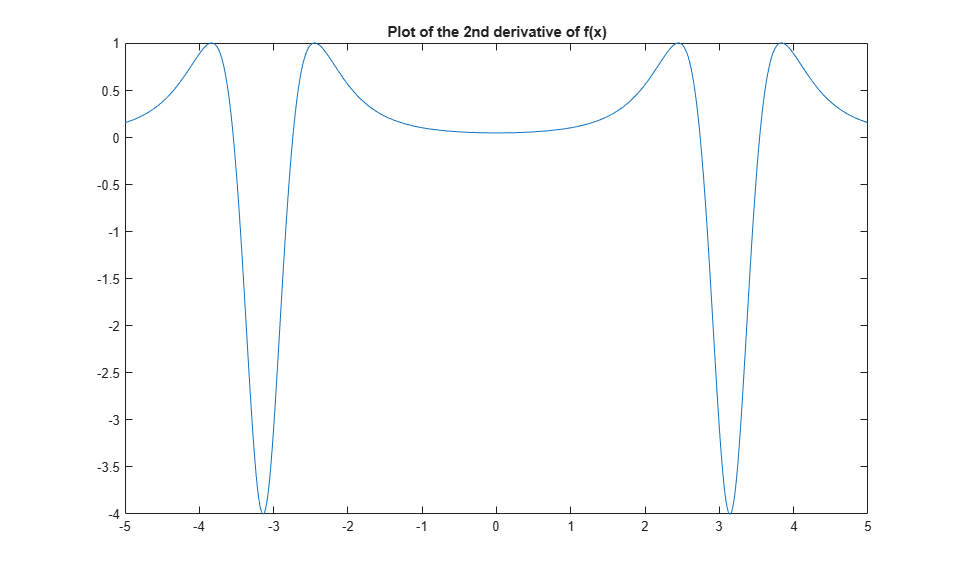The `int` function integrates functions of symbolic variables. The following example shows an attempt to retrieve the original function by integrating the second derivative twice.

```disp('Retrieve the original function by integrating the second derivative twice. Plot the result.') disp('>> g = int(int(f2))') disp('>> fplot(g)') g = int(int(f2)) figure; fplot(g) title("Plot of int(int(f2))") ```
```Retrieve the original function by integrating the second derivative twice. Plot the result. >> g = int(int(f2)) >> fplot(g) g(x) = -8/(tan(x/2)^2 + 9) ```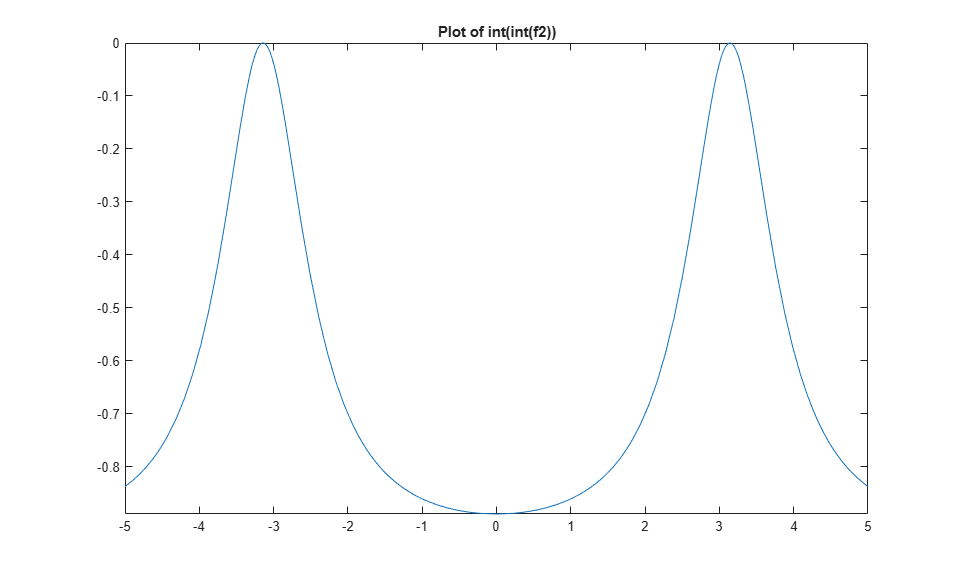Initially, the plots for `f` and `g` look the same. However, their formulas and the y-axis ranges are different.

```disp('Observe the formulas and ranges on the y-axis when comparing f and g') disp('>> subplot(1,2,1)') disp('>> fplot(f)') disp('>> subplot(1,2,2)') disp('>> fplot(g)') disp(' ') figure; subplot(1,2,1) fplot(f) title("Plot of f") subplot(1,2,2) fplot(g) title("Plot of g") ```
```Observe the formulas and ranges on the y-axis when comparing f and g >> subplot(1,2,1) >> fplot(f) >> subplot(1,2,2) >> fplot(g) ```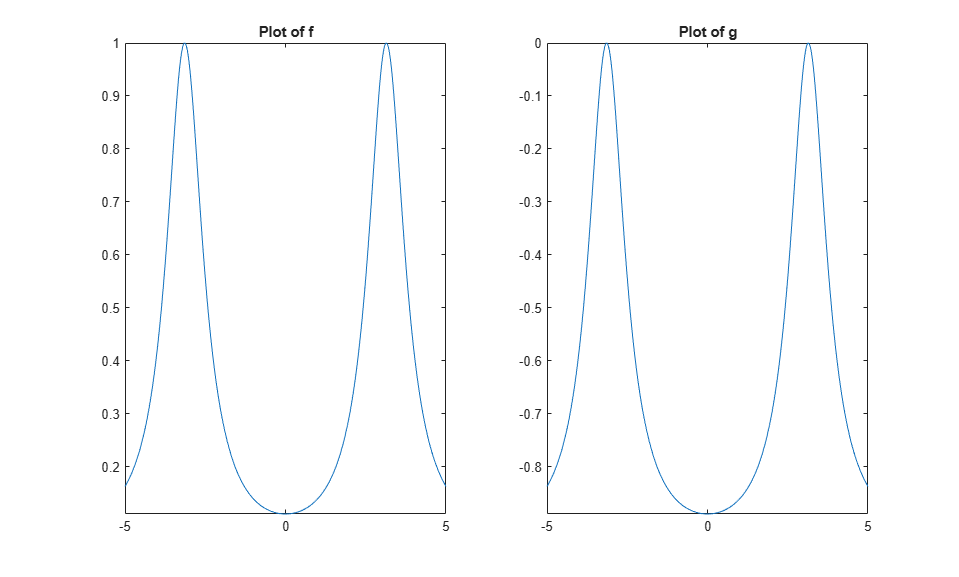The value `e` is the difference between `f` and `g`. It has a complicated formula, but its graph looks like a constant.

```disp('Compute the difference between f and g') disp('>> e = f - g') e = f - g ```
```Compute the difference between f and g >> e = f - g e(x) = 8/(tan(x/2)^2 + 9) + 1/(4*cos(x) + 5) ```

To show that the difference truly is a constant, simplify the equation.

```disp('Simplify the equation to show that the difference between f and g is constant') disp('>> simplify(e)') e = simplify(e) ```
```Simplify the equation to show that the difference between f and g is constant >> simplify(e) e(x) = 1 ```